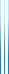# Talks

## Bounded indistinguishability for simple sources

Let $X,Y$ be $\mathrm{polylog}(n)$-indistinguishable. Braverman famously showed that if $Y$ is uniform then $X,Y$ fool $\mathsf{AC^0}$. In contrast, there exists a pair of $\Theta(\sqrt{n})$-indistinguishable distributions which do not even fool OR.

Under what conditions on $X,Y$ can we recover a Braverman-style theorem?

Joint work with Andrej Bogdanov, K. Dinesh, Yuval Ishai, Avi Kaplan, and Akshay Srinivasan.

## AND testing

Linearity testing states that if $\Pr[f(x \oplus y) = f(x) \oplus f(y)] \approx 1$ then $f \approx \mathsf{XOR}$.

Together with Noam Lifshitz, Dor Minzer and Elchanan Mossel, we showed that if $\Pr[f(x \land y) = f(x) \land f(y)] \approx 1$ then $f \approx \mathsf{AND}$.

Together with Gilad Chase, Dor Minzer and Nitin Saurabh, we extended this for arbitrary inner functions (replacing $\oplus$ or $\land$).

## Twenty (simple) questions

Based on our work with Yuval Dagan, Ariel Gabizon and Shay Moran, and on our work with Yuval Dagan, Daniel Kane and Shay Moran.

## Limitations of the Coppersmith–Winograd technique

Based on our work together with Andris Ambainis and François Le Gall.

## Monotone submodular maximization via non-oblivious local search

Maximizing a monotone submodular function over a matroid constraint is a fundamental combinatorial optimization problem, generalizing problems such as Maximum Coverage and MAX-SAT.

Calienscu, Chekuri, Pál and Vondrák devised the continuous greedy algorithm, which gives the optimal $1-1/e$ approximation ratio.

Together with Justin Ward, we have come up with an alternative algorithm which is more combinatorial in nature. We first devised an algorithm for the special case of maximum coverage, and then extended it to the general case.

## Triangle-intersecting families of graphs

An exposition of Triangle-intersecting families of graphs, our joint work with David Ellis and Ehud Friedgut, in which we show that if $\mathcal{F}$ is a family of graphs on $n$ points such that any two graphs in $\mathcal{F}$ contain a triangle, then $\mathcal{F}$ contains at most $1/8$ of the graphs.

The proof uses the spectral approach of Hoffman and Lovász.